SYLLABUS  Previous: 3.1 Mathematical background  Up: 3 FINITE ELEMENT METHOD  Next: 3.3 Numerical quadrature

## 3.2 An engineer's formulation

Slide : [ Weak form - By parts - time - space - Ax=b || VIDEO login]

After a section on what a physicist believes is a mathematician's view of the subject, it is time for an example. Using the format of a recipe'' that is applicable to a broad class of practical problems, we show here how the advection-diffusion equation (1.3.2#eq.2) is discretized using Galerkin linear finite elements (FEMs) and how it is implemented.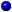Derive a weak variational form.
Multiply'' your equation by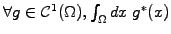where the complex conjugate is necessary only if your equation(s) is (are) complex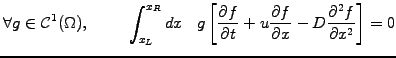(1)Integrate by parts,
so as to avoid having to use quadratic basis functions to discretize the second order diffusion operator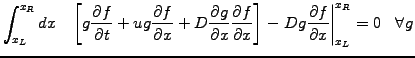(2)

Assuming a periodic domain, the surface term has here been canceled, imposing natural boundary conditions.Discretize time
with a finite difference backward in time and using a partially implicit evaluation of the unknown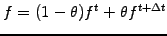where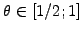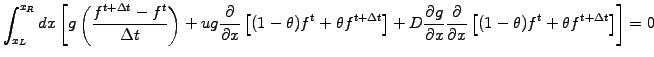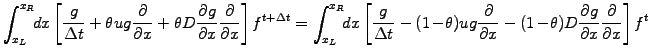(3)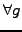. All the unknowns have been reassembled on the left. Re-scale by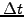, andDiscretize space
using linear roof-tops and a Galerkin choice for the test functions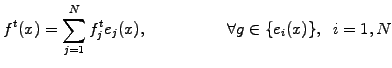(4)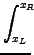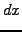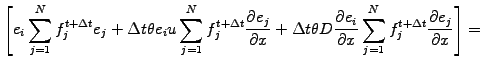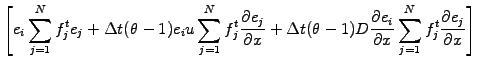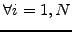(5)

Note how the condition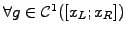is here used to create as many independent equations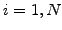as there are unknowns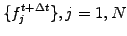. All the essential boundary conditions are then imposed by allowing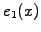and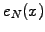to overlap in the periodic domain. Since only the basis and test functions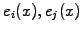and perhaps the problem coefficients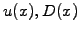remain space dependent, the discretized equations are all written in terms of inner products, involving overlap integrals of the form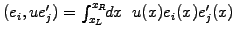. Reassembling them all in a matrix,Write a linear system
through which the unknown values from the next time step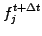can implicitly be obtained from the current values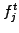by solving a linear system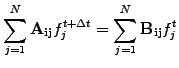(6)

To relate the Galerkin linear FEM scheme (3.2#eq.5) with the code that has been implemented in the JBONE applet, it is necessary now to evaluate the overlap integrals. This is usually performed numerically using a quadrature. In the case of a homogeneous mesh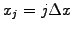, a constant advection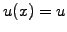and diffusion coefficient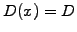, the coming section shows how explicit expressions can be obtained from the same rules to define directly the matrix elements.

SYLLABUS  Previous: 3.1 Mathematical background  Up: 3 FINITE ELEMENT METHOD  Next: 3.3 Numerical quadrature